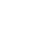Sharing is caring!

In subnetting the only thing you really need to memorize is…..
And remember the number 256
Learn that and everything else is easy…..
Now let’s learn how to find the number of IP addresses for a subnet mask or CIDR notation.
Let’s say you want to know how many IP address you have given a subnet mask.
Example 1:
Take 224 and subtract that from 256…. So 256 – 224 is 32, so that means you have 32 IP addresses available for the mask of 255.255.255.224. Now to find the assignable addresses you subtract 2 (one for the broadcast address and one for the network address) so you have 30 assignable IP addresses. Simple right?
Now let’s say they give you this mask, /29, well here is where that little memorization comes in. So let’s convert that to dotted decimal…. 255.255.255.248
Take 248 and subtract that from 256….. So 256 – 248 is 8, so that means you have 8 IP addresses available for the mask of 255.255.255.248 or /29, which in turn means there are 6 assignable IP addresses after you subtract the broadcast and network IP addresses. Easy right?
Example 2:
255.255.240.0…… Now what! Well we just have to do one more thing.
Let’s use that .240. 0 and pretend the .0 does not exist so just think .240, now from the above work figure out the number of IP addresses for .240 which we would get by subtracting that from 256, remember? So 256 – 240 is 16. Now here is the “one more thing” you need to do. Take that 16 and multiply it by 256 (we use 256 because we are one octet up). So 256*16 is 4,096, and there you have the number of IP address available for the mask of 255.255.240.0 and just subtract the broadcast and network addresses and you have 4,094 assignable IP addresses.
Example 3:
255.192.0.0……Now what! Well we just have to do two more things.
Let’s use that .192.0. 0 and pretend the .0.0 does not exist so just think .192, now from the above work, figure out the number of IP addresses for .192 which we would get by subtracting that from 256, remember? So 256 – 192 is 64. Now here is the “two more things” you need to do. First take 256*256 (we do 256 twice because we are 2 octets up) which is 65,536 now take 65,536 and multiply it by the 64. So 65,536*64 is 4,194,304, and there you have the number of IP address available for the mask of 255.192.0.0 and just subtract the broadcast and network addresses and you have 4,194,302 assignable IP addresses.
Example 4:
248.0.0.0……Now what! Well we just have to do three more things.
For this we have to use 248.0.0.0 and then pretend the .0.0.0 does not exist so just think .248, now from the above work, figure out the number of IP addresses for .248 which we would get by subtracting that from 256, So 256 – 248 is 8. Now here is the “three more things” you need to do. First take 256*256 then take that result and multiply it by 256 (we do 256 three times because we are 3 octets up) which 256*256 is 65,536 now take 65,536 and multiply it by 256 and you get 16,777,216. Now you take 16,777,216 and multiply it by 8 from above and you get 134,217,728 and there you have the number of IP address available for the mask of 248.0.0.0 and just subtract the broadcast and network addresses and you have 134,217,728 assignable IP addresses.

Remember this, substitute all 255’s with 0’s and all 0’s with 255’s.

We will use the above four examples.
Example 1:
255.255.255.224 with 32 IP Addresses. To find the wildcard mask remember replace the 255’s with 0’s, now take the number of IP addresses and subtract 1 and place that where the 224 is. So you would get, 0.0.0.31, and there is your wildcard mask.
Example 2:
255.255.240.0 with 16 IP addresses. Remember substitute all 255’s with 0’s and all 0’s with 255’s so you have this 0.0.?.255 and the ? would be 16 – 1 which is 15. So you get a wildcard mask of 0.0.15.255, not bad right.
Example 3:
255.192.0.0 with 64 IP addresses. Now with the substitutions and subtract 1 from the IP addresses you get a wildcard mask of 0.63.255.255, you’re getting it, one more to go.
Example 4:
248.0.0.0 with 8 IP addresses. Again with the substitutions and subtract 1 from the IP addresses you get a wildcard mask of 7.255.255.255, now you got it, wildcard masking made easy.

:::::Ref &  Credit::: http://www.aelius.com/njh/subnet_sheet.html

## One comment

1.Engr Mohsin says:

Kamaal

This site uses Akismet to reduce spam. Learn how your comment data is processed.